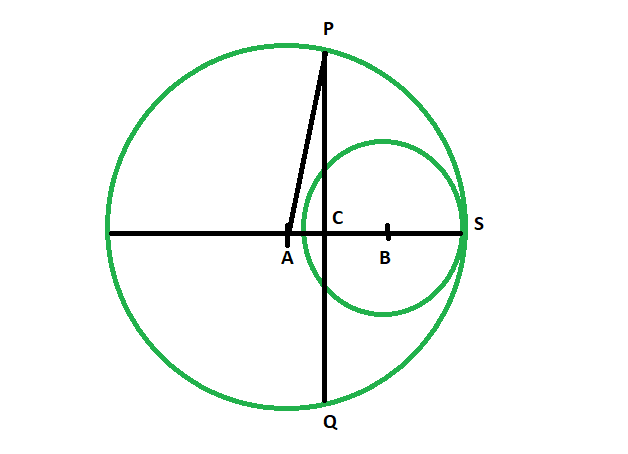# Length of the perpendicular bisector of the line joining the centers of two circles

Given are two circles whose radii are given, such that the smaller lies completely within the bigger circle, and they touch each other at one point. We have to find the length of the perpendicular bisector of the line joining the centres of the circles.

Examples:

```Input: r1 = 5, r2 = 3
Output: 9.79796

Input: r1 = 8, r2 = 4
Output: 15.4919
```Approach:

• Let the two circles have center at A and B.The perpendicular bisector PQ, bisects the line at C.
• Let radius of bigger circle = r1
radius of smaller circle = r2
• so, AB = r1-r2,
• therefore, AC = (r1-r2)/2
• In, the figure, we see
PA = r1
• in triangle ACP,
PC^2 + AC^2 = PA^2
PC^2 = PA^2 – AC^2
PC^2 = r1^2 – (r1-r2)^2/4
• so, PQ = 2*√(r1^2 – (r1-r2)^2/4)

Length of the perpendicular bisector = 2 * sqrt(r1^2 – (r1-r2)*(r1-r2)/4)

Below is the implementation of the above approach:

## C++

 `// C++ program to find the Length ` `// of the perpendicular bisector ` `// of the line joining the centers ` `// of two circles in which one lies ` `// completely inside touching the ` `// bigger circle at one point ` ` `  `#include ` `using` `namespace` `std; ` ` `  `void` `lengperpbisect(``double` `r1, ``double` `r2) ` `{ ` `    ``double` `z = 2 * ``sqrt``((r1 * r1) ` `                        ``- ((r1 - r2) ` `                           ``* (r1 - r2) / 4)); ` ` `  `    ``cout << ``"The length of the "` `         ``<< ``"perpendicular bisector is "` `         ``<< z << endl; ` `} ` ` `  `// Driver code ` `int` `main() ` `{ ` `    ``double` `r1 = 5, r2 = 3; ` `    ``lengperpbisect(r1, r2); ` `    ``return` `0; ` `} `

## Java

 `// Java program to find the Length ` `// of the perpendicular bisector ` `// of the line joining the centers ` `// of two circles in which one lies ` `// completely inside touching the ` `// bigger circle at one point ` ` `  `class` `GFG { ` `     `  `static` `void` `lengperpbisect(``double` `r1, ``double` `r2) ` `{ ` `    ``double` `z = ``2` `* Math.sqrt((r1 * r1) ` `                        ``- ((r1 - r2) ` `                        ``* (r1 - r2) / ``4``)); ` ` `  `    ``System.out.println(``"The length of the "` `        ``+ ``"perpendicular bisector is "` `        ``+ z ); ` `} ` ` `  `// Driver code ` `public` `static` `void` `main(String[] args)  ` `{ ` `    ``double` `r1 = ``5``, r2 = ``3``; ` `    ``lengperpbisect(r1, r2); ` `} ` `} ` ` `  `// This code has been contributed by 29AjayKumar `

## Python3

 `     `  `# Python program to find the Length ` `# of the perpendicular bisector ` `# of the line joining the centers ` `# of two circles in which one lies ` `# completely inside touching the ` `# bigger circle at one point ` ` `  `def` `lengperpbisect(r1, r2): ` `    ``z ``=` `2` `*` `(((r1 ``*` `r1) ``-` `((r1 ``-` `r2) ``*` `(r1 ``-` `r2) ``/` `4``))``*``*``(``1``/``2``)); ` ` `  `    ``print``(``"The length of the perpendicular bisector is "``, z); ` ` `  ` `  `# Driver code ` `r1 ``=` `5``; r2 ``=` `3``; ` `lengperpbisect(r1, r2); ` ` `  `# This code contributed by PrinciRaj1992  `

## C#

 `// C# program to find the Length ` `// of the perpendicular bisector ` `// of the line joining the centers ` `// of two circles in which one lies ` `// completely inside touching the ` `// bigger circle at one point ` `using` `System; ` ` `  `class` `GFG  ` `{ ` `     `  `static` `void` `lengperpbisect(``double` `r1, ``double` `r2) ` `{ ` `    ``double` `z = 2 * Math.Sqrt((r1 * r1) ` `                        ``- ((r1 - r2) ` `                        ``* (r1 - r2) / 4)); ` ` `  `    ``Console.WriteLine(``"The length of the "` `        ``+ ``"perpendicular bisector is "` `        ``+ z ); ` `} ` ` `  `// Driver code ` `public` `static` `void` `Main()  ` `{ ` `    ``double` `r1 = 5, r2 = 3; ` `    ``lengperpbisect(r1, r2); ` `} ` `} ` ` `  `// This code has been contributed by anuj_67.. `

Output:

```The length of the perpendicular bisector is 9.79796
```

My Personal Notes arrow_drop_upCheck out this Author's contributed articles.

If you like GeeksforGeeks and would like to contribute, you can also write an article using contribute.geeksforgeeks.org or mail your article to contribute@geeksforgeeks.org. See your article appearing on the GeeksforGeeks main page and help other Geeks.

Please Improve this article if you find anything incorrect by clicking on the "Improve Article" button below.

Article Tags :
Practice Tags :

Be the First to upvote.

Please write to us at contribute@geeksforgeeks.org to report any issue with the above content.